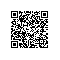# [剑指Offer] 第4章课后题详解

## 二叉树的镜像

struct BinaryTreeNode{
int             m_nValue;
BinaryTreeNode* m_pLeft;
BinaryTreeNode* m_pRight;
};

### 分析### 解法

//使用容器适配器queue，《剑指Offer》中第23题用的是deque实现层次遍历，但个人觉得不需要，deque的双端进出和随机访问都没用到，这里只需要一个能实现先进先出的容器就足够了。
#include <queue>

auto MirrorRecursively(BinaryTreeNode *pRoot) -> void{

//处理空树
if(pRoot == NULL){
return;
}

queue<BinaryTreeNode*> queueTreeNode;
//将根节点加入到队列中
queueTreeNode.push(pRoot);

while(!queueTreeNode.empty()){
//取出队头的节点
BinaryTreeNode *pNode = queueTreeNode.front();
queueTreeNode.pop();

//其实不加此判定也可以正常运行，叶节点的左右子节点都为空，进行交换后也不会有任何影响，只是会多进行几次操作，在叶节点数量较大时可能会对算法性能造成影响。
if(pNode -> m_pLeft == NULL && pNode -> m_pRight == NULL){
continue;
}

//如果左子树不为空，将左子树的根节点加入到队列中
if(pNode -> m_pLeft){
queueTreeNode.push(pNode -> m_pLeft);
}

//如果右子树不为空，将右子树的根节点加入到队列中
if(pNode -> m_pRight){
queueTreeNode.push(pNode -> m_pRight);
}

//交换左右子树
BinaryTreeNode* tmp = pNode -> m_pLeft;
pNode -> m_pLeft = pNode -> m_pRight;
pNode -> m_pRight = tmp;
}
}


## 判断前序遍历

### 解法

auto VerifySquenceOfBST(const int sequence[], const unsigned length) -> bool{

//这里原书类似的判断后续遍历的算法返回的是false，但个人觉得应该是返回true，根据搜索二叉树定义，空树也是二叉搜索树！
if(sequence == NULL || length == 0){
return true;
}

//数组的第一个元素为根节点的值
int root = sequence;

//从第二个元素开始，依次寻找第一个大于根节点值的元素
int i = 1;
for(;i < length ; ++i){
if(sequence[i] > root){
break;
}
}

//判断根节点右子树上的所有节点的值是否都大于根节点的值
int j = i;
for(;j < length ; ++j){
if(sequence[j] < root){
return false;
}
}

//如果左子树不为空，递归判定左子树是否为二叉搜索树
bool left = true;
if(i > 1){
left = VerifySquenceOfBST(sequence, i - 1);
}

//如果右子树不为空，递归判定右子树是否为二叉搜索树
bool right = true;
if(i < length){
right = VerifySquenceOfBST(sequence + i, length - i);
}

return (left && right);
}

## 字符的组合

### 解答

auto groupOfChars(string s, int strLength) -> void{
//依次求长度从1~字符串长度的所有组合
for(int i = 1; i <= strLength; ++i){
groups(s, strLength, i, "");
}
}

//参数分别为：字符串，字符串长度，组合长度，头字符串（累计存储的第一个字符）
auto groups(string s, int strLength, int groupLength, string headStr) -> void{

//如果字符串长度等于组合长度，则输出字符串（头字符串放前面）
if(strLength == groupLength){
cout << headStr << s << endl;
return;
}

//如果组合长度为1，则依次输出字符串中的单个字符（头字符串放前面）
if(groupLength == 1){
for(int i = 0; i < strLength; ++i){
cout << headStr << s[i] << endl;
}
return;
}

//将除第一个字符外的字符串截取出来
string sub(s.begin() + 1, s.end());
//不包含第一个字符的情况，头字符串不做改动，依旧为之前递归下来的头字符串。
groups(sub, strLength - 1, groupLength, headStr);
//包含第一个字符的情况，把字符串中的第一个字符截取出来，加到之前递归下来的头字符串之后
groups(sub, strLength - 1, groupLength - 1, headStr);
}

## 正方体的顶点### 解法

//设置一个全局变量来存储是否存在组合
bool flag = false;
//接收维度固定为8的数组，这样如果传入数组元素不足8个会自动补0，也可以手动传入数组长度，更便于拓展
auto func(int numbers) -> bool{
allArray(numbers, 0);
return flag;
}
auto canBeCube(int numbers) -> bool{
bool flag1 = numbers+numbers+numbers+numbers == numbers+numbers+numbers+numbers;
bool flag2 = numbers+numbers+numbers+numbers == numbers+numbers+numbers+numbers;
bool flag3 = numbers+numbers+numbers+numbers == numbers+numbers+numbers+numbers;

//只要有任意一个排列符合要求就将全局变量flag设置为真
if(flag1 && flag2 && flag3){
flag = true;
}
return (flag1 && flag2 && flag3);
}

auto allArray(int numbers, int begin) -> void{
//本算法将所有符合要求的排列都求出来了，题目要求只需要判定是否存在符合要求的排列，如果只是完成题目要求可以在这里加上，如果全局变量flag为真，直接返回。
//达到数组末尾，判定当前排列是否符合条件，符合的话就打印出来。
if(begin >= 8){
if(!canBeCube(numbers)) return;
for(int i = 0; i < 8; ++i){
cout << numbers[i] << " ";
}
cout << endl;
return;
}

//依次将数组中的元素与首元素进行交换，完成递归后，再交换回来
for(int i = begin;i < 8; ++i){
int tmp = numbers[i];
numbers[i] = numbers[begin];
numbers[begin] = tmp;

allArray(numbers, begin + 1);

tmp = numbers[i];
numbers[i] = numbers[begin];
numbers[begin] = tmp;
}
}

## 8个皇后

### 解法

//用于保存符合条件排列个数的全局变量
int sum = 0;
auto canPlace(int numbers) -> bool{
for(int i = 0; i < 8; ++i){
for(int j = 0; j < 8; ++j){
//如果是同一个棋子，则跳过
if(i == j){
continue;
}
//如果有在同一对角线上的棋子，直接返回false
if(i - j ==  numbers[i] - numbers[j] || j - i == numbers[i] - numbers[j])
{
return false;
}
}
}
//排列数加1
++sum;
return true;
}使用钉钉扫一扫加入圈子
+ 订阅